# Unprojecting a Digital Elevation Model (DEM)

This example shows how to convert a USGS DEM into a regular latitude-longitude grid having comparable spatial resolution. U.S. Geological Survey (USGS) 30-meter Digital Elevation Models (DEMs) are regular grids (raster data) that use the UTM coordinate system. Using such DEMs in applications may require reprojecting and resampling them. You can readily apply the approach show here to projected map coordinate systems other than UTM and to other DEMs and most types of regular data grids.

First, set the output display format to `longG` so that the output displays more decimal places. Get the current output format so that you can restore it at the end of the example.

```currentFormat = get(0,'format'); format longG ```

### Step 1: Import the DEM and its Metadata

This example uses a USGS DEM for a quadrangle 7.5-arc-minutes square located in the White Mountains of New Hampshire, USA. Import the data and a map cells reference object using the `readgeoraster` function. Get additional metadata using the `georasterinfo` function.

```[Z,R] = readgeoraster('MtWashington-ft.grd','OutputType','double'); info = georasterinfo('MtWashington-ft.grd'); ```

Replace missing data with `NaN` values.

```m = info.MissingDataIndicator; Z = standardizeMissing(Z,m); ```

### Step 2: Get Projection Information

Get information about the projected coordinate reference system by querying the `ProjectedCRS` property of the reference object. The result is a `projcrs` object. Then, get the ellipsoid for the coordinate reference system.

```p = R.ProjectedCRS; ellipsoid = p.GeographicCRS.Spheroid ```
```ellipsoid = referenceEllipsoid with defining properties: Code: 7008 Name: 'Clarke 1866' LengthUnit: 'meter' SemimajorAxis: 6378206.4 SemiminorAxis: 6356583.8 InverseFlattening: 294.978698213898 Eccentricity: 0.0822718542230038 and additional properties: Flattening ThirdFlattening MeanRadius SurfaceArea Volume ```

### Step 3: Determine which UTM Zone to Use and Construct a Map Axes

From the `Name` property of the `projcrs` object, you can tell that the DEM is gridded in a Universal Transverse Mercator (UTM) coordinate system.

```p.Name ```
```ans = "UTM Zone 19, Northern Hemisphere" ```

To find the UTM zone, first locate the center of the DEM in UTM coordinates. Then, convert the coordinates to latitude-longitude.

```[M,N] = size(Z); xCenterIntrinsic = (1 + N)/2; yCenterIntrinsic = (1 + M)/2; [xCenter,yCenter] = intrinsicToWorld(R,xCenterIntrinsic,yCenterIntrinsic); [latCenter,lonCenter] = projinv(p,xCenter,yCenter) ```
```latCenter = 44.3124367104673 lonCenter = -71.3126432693478 ```

Find the UTM zone for the DEM by using the `utmzone` function.

```utmZone = utmzone(latCenter,lonCenter) ```
```utmZone = '19T' ```

Use the zone and ellipsoid to create a map axes.

```figure axesm('utm','zone',utmZone,'geoid',ellipsoid) axis off gridm mlabel on plabel on framem on ```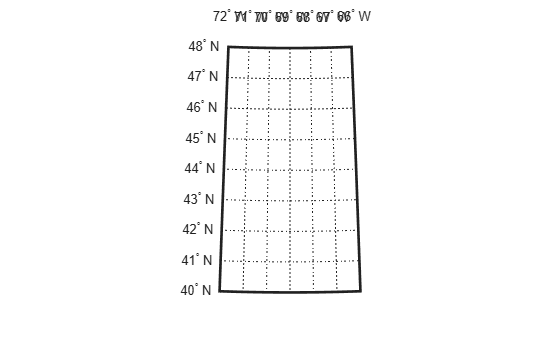Note: if you can visually place the approximate location of New Hampshire on a world map, then you can confirm this result with the `utmzoneui` GUI.

` utmzoneui(actualZone)`

### Step 4: Display the Original DEM on the Map Axes

Use `mapshow` (rather than `geoshow` or `meshm`) to display the DEM on the map axes because the data are gridded in map (x-y) coordinates.

```mapshow(Z,R,'DisplayType','texturemap') demcmap(Z) ```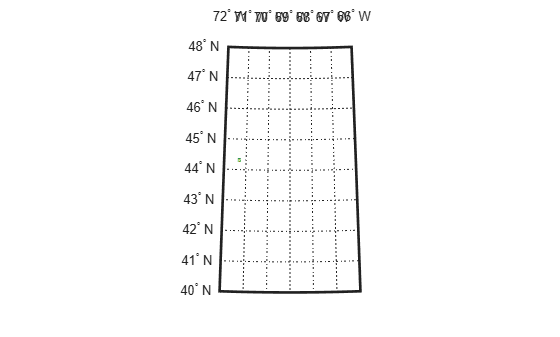The DEM covers such a small part of this map that it may be hard to see (look between 44 and 44 degrees North and 72 and 71 degrees West), because the map limits are set to cover the entire UTM zone. You can reset them (as well as the map grid and label parameters) to get a closer look.

```setm(gca,'MapLatLimit',[44.2 44.4],'MapLonLimit',[-71.4 -71.2]) setm(gca,'MLabelLocation',0.05,'MLabelRound',-2) setm(gca,'PLabelLocation',0.05,'PLabelRound',-2) setm(gca,'PLineLocation',0.025,'MLineLocation',0.025) ```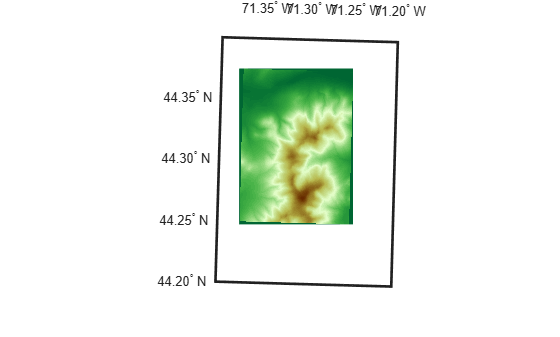When it is viewed at this larger scale, narrow wedge-shaped areas of uniform color appear along the edge of the grid. These are places where `Z` contains the value NaN, which indicates the absence of actual data. By default they receive the first color in the color table, which in this case is dark green. These null-data areas arise because although the DEM is gridded in UTM coordinates, its data limits are defined by a latitude-longitude quadrangle. The narrow angle of each wedge corresponds to the non-zero "grid declination" of the UTM coordinate system in this part of the zone. (Lines of constant x run precisely north-south only along the central meridian of the zone. Elsewhere, they follow a slight angle relative to the local meridians.)

### Step 5: Define the Output Latitude-Longitude Grid

The next step is to define a regularly-spaced set of grid points in latitude-longitude that covers the area within the DEM at about the same spatial resolution as the original data set.

First, you need to determine how the latitude changes between rows in the input DEM (i.e., by moving northward by 30 meters).

```rng = R.CellExtentInWorldY; % In meters, consistent with p.LengthUnit latcrad = deg2rad(latCenter); % latCenter in radians % Change in latitude, in degrees dLat = rad2deg(meridianfwd(latcrad,rng,ellipsoid) - latcrad) ```
```dLat = 0.000269984939366415 ```

The actual spacing can be rounded slightly to define the grid spacing to be used for the output (latitude-longitude) grid.

```gridSpacing = 1/4000; % In other words, 4000 samples per degree ```

To set the extent of the output (latitude-longitude) grid, start by finding the corners of the DEM in UTM map coordinates.

```xCorners = R.XWorldLimits([1 1 2 2])' yCorners = R.YWorldLimits([1 2 2 1])' ```
```xCorners = 310380 310380 320730 320730 yCorners = 4901880 4916040 4916040 4901880 ```

Then convert the corners to latitude-longitude. Display the latitude-longitude corners on the map (via the UTM projection) to check that the results are reasonable.

```[latCorners, lonCorners] = projinv(p,xCorners, yCorners) hCorners = geoshow(latCorners,lonCorners,'DisplayType','polygon',... 'FaceColor','none','EdgeColor','red'); ```
```latCorners = 44.2474175605687 44.3747915486804 44.3774240601986 44.2500384686392 lonCorners = -71.3749065609587 -71.3800513603087 -71.2502438233865 -71.2453790282992 ```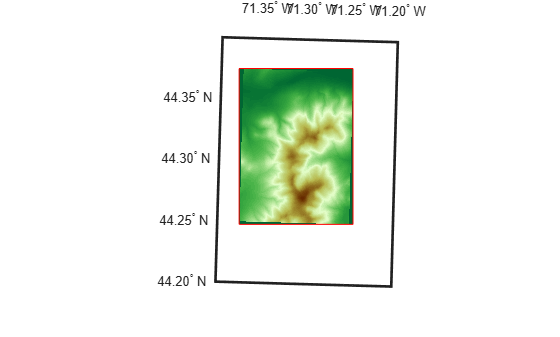Next, round outward to define an output latitude-longitude quadrangle that fully encloses the DEM and aligns with multiples of the grid spacing.

```latSouth = gridSpacing * floor(min(latCorners)/gridSpacing) lonWest = gridSpacing * floor(min(lonCorners)/gridSpacing) latNorth = gridSpacing * ceil( max(latCorners)/gridSpacing) lonEast = gridSpacing * ceil( max(lonCorners)/gridSpacing) qlatlim = [latSouth latNorth]; qlonlim = [lonWest lonEast]; dlat = 100*gridSpacing; dlon = 100*gridSpacing; [latquad, lonquad] = outlinegeoquad(qlatlim, qlonlim, dlat, dlon); hquad = geoshow(latquad, lonquad, ... 'DisplayType','polygon','FaceColor','none','EdgeColor','blue'); snapnow; ```
```latSouth = 44.24725 lonWest = -71.38025 latNorth = 44.3775 lonEast = -71.24525 ```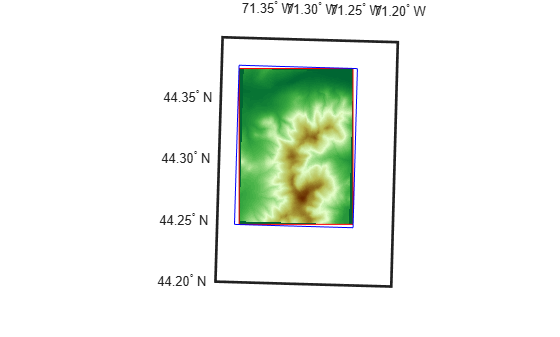Finally, construct a geographic raster referencing object for the output grid. It supports transformations between latitude-longitude and the row and column subscripts. In this case, use of a world file matrix, W, enables exact specification of the grid spacing.

```W = [gridSpacing 0 lonWest + gridSpacing/2; ... 0 gridSpacing latSouth + gridSpacing/2] ```
```W = 0.00025 0 -71.380125 0 0.00025 44.247375 ```
```nRows = round((latNorth - latSouth) / gridSpacing) nCols = round(wrapTo360(lonEast - lonWest) / gridSpacing) ```
```nRows = 521 nCols = 540 ```
```Rlatlon = georasterref(W,[nRows nCols],'cells'); Rlatlon.GeographicCRS = p.GeographicCRS ```
```Rlatlon = GeographicCellsReference with properties: LatitudeLimits: [44.24725 44.3775] LongitudeLimits: [-71.38025 -71.24525] RasterSize: [521 540] RasterInterpretation: 'cells' ColumnsStartFrom: 'south' RowsStartFrom: 'west' CellExtentInLatitude: 1/4000 CellExtentInLongitude: 1/4000 RasterExtentInLatitude: 0.13025 RasterExtentInLongitude: 0.135 XIntrinsicLimits: [0.5 540.5] YIntrinsicLimits: [0.5 521.5] CoordinateSystemType: 'geographic' GeographicCRS: [1x1 geocrs] AngleUnit: 'degree' ```

`Rlatlon` fully defines the number and location of each cell in the output grid.

### Step 6: Map Each Output Grid Point Location to UTM X-Y

Finally, you're ready to make use of the map projection, applying it to the location of each point in the output grid. First compute the latitudes and longitudes of those points, stored in 2-D arrays.

```[rows,cols] = ndgrid(1:nRows, 1:nCols); [lat,lon] = intrinsicToGeographic(Rlatlon,cols,rows); ```

Then apply the projection to each latitude-longitude pair, arrays of UTM x-y locations having the same shape and size as the latitude-longitude arrays.

```[XI,YI] = projfwd(p,lat,lon); ```

At this point, `XI(i,j)` and `YI(i,j)` specify the UTM coordinate of the grid point corresponding to the i-th row and j-th column of the output grid.

### Step 7: Resample the Original DEM

The final step is to use the MATLAB `interp2` function to perform bilinear resampling.

At this stage, the value of projecting from the latitude-longitude grid into the UTM map coordinate system becomes evident: it means that the resampling can take place in the regular X-Y grid, making `interp2` applicable. The reverse approach, unprojecting each (X,Y) point into latitude-longitude, might seem more intuitive but it would result in an irregular array of points to be interpolated -- a much harder task, requiring use of the far more costly `griddata` function or some rough equivalent.

```[rows,cols] = ndgrid(1:M,1:N); [X,Y] = intrinsicToWorld(R,cols,rows); method = 'bilinear'; extrapval = NaN; Zlatlon = interp2(X,Y,Z,XI,YI,method,extrapval); ```

View the result in the projected axes using `geoshow`, which will re-project it on the fly. Notice that it fills the blue rectangle, which is aligned with lines of latitude and longitude. (In contrast, the red rectangle, which outlines the original DEM, aligns with UTM x and y.) Also notice NaN-filled regions along the edges of the grid. The boundaries of these regions appear slightly jagged, at the level of a single grid spacing, due to round-off effects during interpolation. Move the red quadrilateral and blue quadrangle to the top, to ensure that they are not hidden by the raster display.

```geoshow(Zlatlon,Rlatlon,'DisplayType','texturemap') uistack([hCorners hquad],'top') ```Restore the original output display format.

```format(currentFormat) ```

### Credits

MtWashington-ft.grd (and supporting files):

``` United States Geological Survey (USGS) 7.5-minute Digital Elevation Model (DEM) for the Mt. Washington quadrangle, with elevation in meters. http://edc.usgs.gov/products/elevation/dem.html```
` For more information, run:`
` >> type MtWashington-ft.txt`